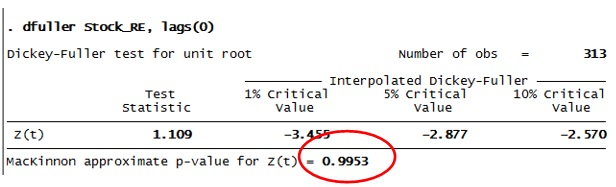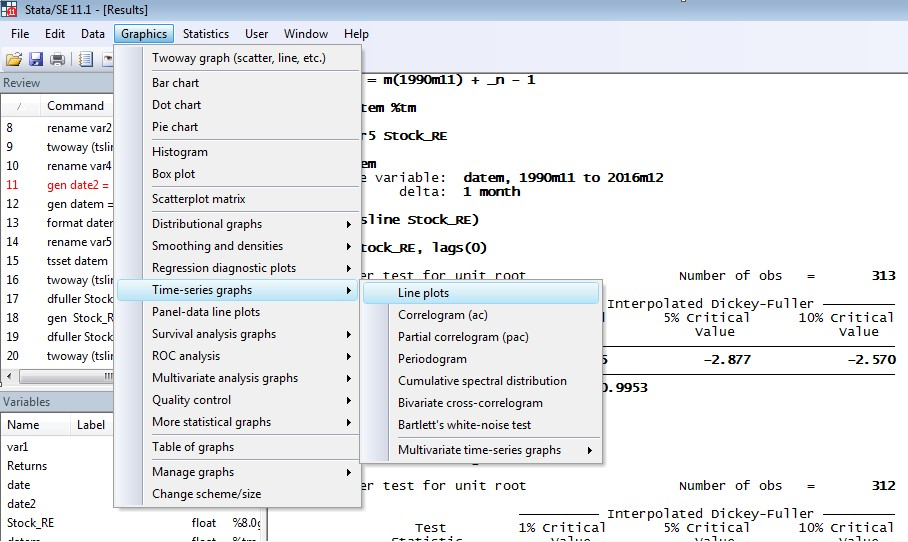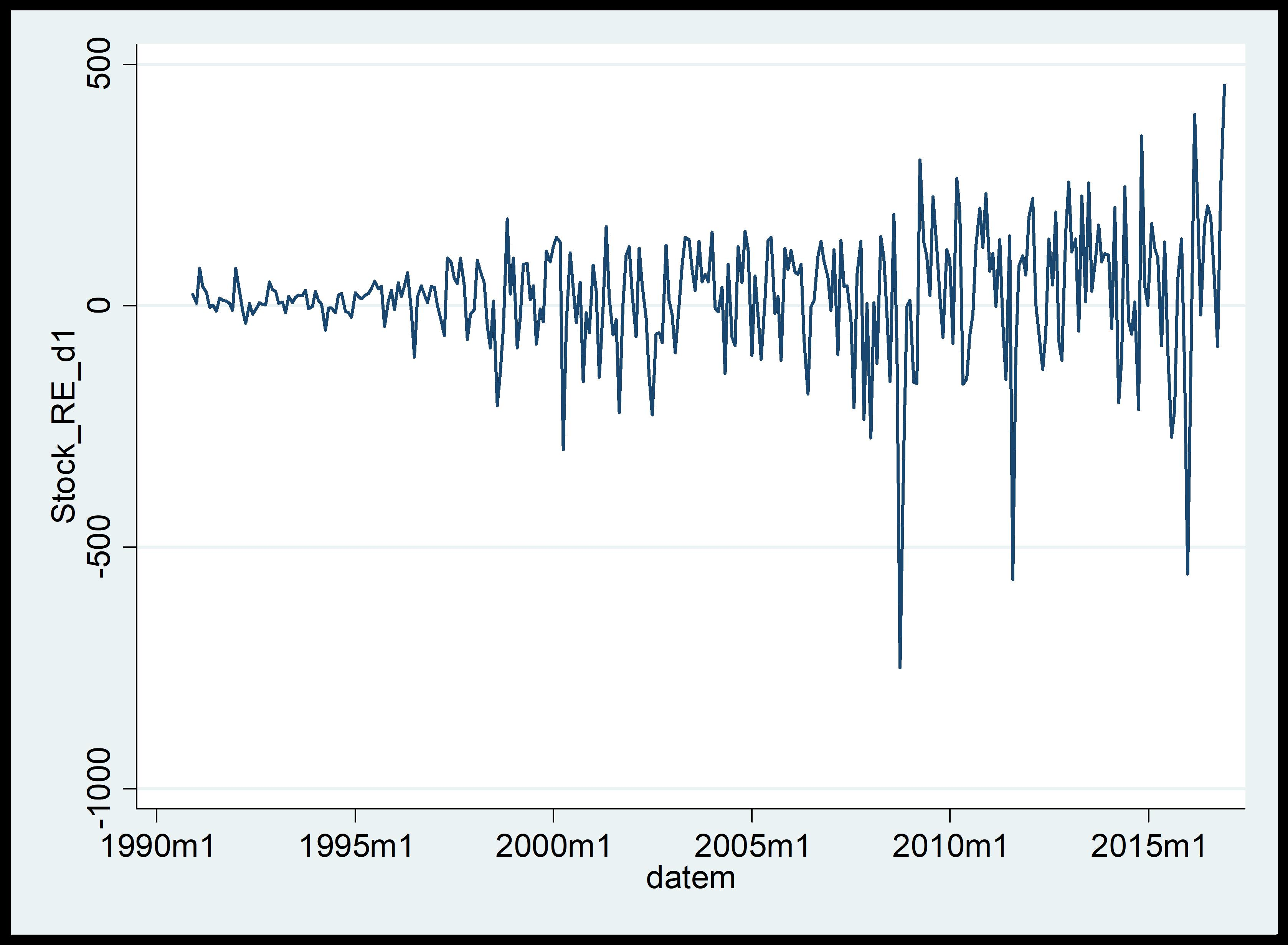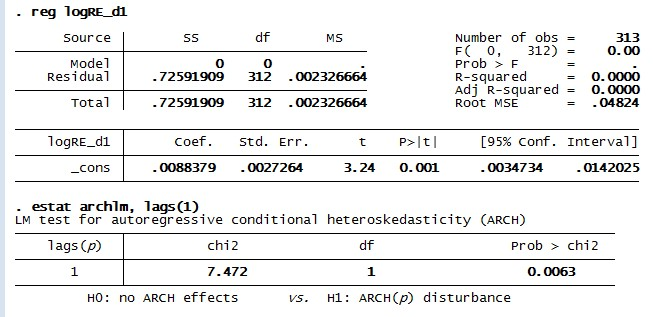# How to identify ARCH effect for time series analysis in STATA?

The previous articles showed how to apply Vector Auto Regression (VAR) and Vector Error Correction Model (VECM) based on the assumption that the variables either have a long run or short run causality among them. Some financial time series such as stock returns show wide swings for an extended period of time. Such behaviour is known as volatility. Volatility only represents a high variability in a series over time. This article explains the issue of volatility in data using Autoregressive Conditional Heteroscedasticity (ARCH) model. It will identify the ARCH effect in a given time series in STATA.

Heteroscedasticity represents the problem of unequal variances over time. Therefore a time series with volatility is termed as having ‘ARCH’ effect. The treatment of ARCH model is slightly different from other models like Auto Regressive Integrated Moving Average (ARIMA), VAR and VECM. In order to demonstrate ARCH model in STATA, this article takes into consideration the stock price data for the period 1990 to 2016.

## Setting the time variable

Firstly set the time variable for the new time series stock returns. This is similar to the process followed for Gross Domestic Product (GDP) in the previous articles. Since the data of 1990-2016 is in monthly format, use the below command:

`gen datem = m(1990m11) + _n - 1`

Here the time series variable is ‘datem’. Then format the time series data of stock returns by using the command:

`format datem %tm`

Lastly, set out time variable “date” as a time series variable by using the command:

`tsset datem`

## Checking stationarity

To start with the analysis first check the stationarity of the stock returns time series through Dickey-Fuller test. The command for this test is:

`dfuller LOGStock_RE, lags(0)`

Here note that variable ‘logStock_RE’ represents time series stock return. As per the results of the test, stock returns are non-stationarity with an insignificant p-value as shown in the figure below.Figure 1: Results of ADF for logRE in STATA

Therefore, the time series stock returns are non-stationary. Thus further analysis is not possible. To transform the time series into stationary, use the method of first differencing. The command for first differencing is:

`gen  logRE_d1 = d1.logRE`

This command reveals the first-differenced time series of stock returns as variable ‘logRE_d1’. Again check the stationarity of first differenced series by using augmented Dickey-Fuller test. Stationarity is present in the series. Therefore proceed to ARCH model.

## Checking the presence of volatility

To start with ARCH model it is imperative to check the presence of volatility in a time series data. The finest way to check is using time series graphs. To plot a time series graph of logRE_d1, follow these steps (figure below):

1. Select ‘Graphics’ on the output window
2. Select ‘Time-series graphs’
3. Click on ‘Line plots’Figure 2: Pathway for time series to identify ARCH effect in STATA

Alternatively, use the below command to generate the graph:

`twoway (tsline logRE_d1)`

The result line plot of the time series ‘Stock_RE_d1’ will appear.Figure 3: Line plot of stock returns to identify ARCH effect in STATA

It is noticeable in the figure above that the first-differenced time series of stock returns are highly volatile. This is because the returns are showing wide swings on some periods and calm in others. Therefore, a case of unequal variance is present here. However, the line graph cannot alone indicate ARCH effect. Therefore, to identify the presence of ARCH effect in time series stock exchange, further perform different steps.

## Check for normality

Similar to confirming stationarity of time series, also confirm the normality of residuals for time series ‘Stock_RE_d1’. To perform the normality, use the command:

`histogram logRE_d1`

OR

• Select ‘Graphics’ on the output window
• Select ‘Histogram’.

The histogram will appear on result window of STATA as shown in the figure below.Figure 4: Normality of stock returns to identify ARCH effect in STATA

These series are leptokurtic. This means that they have lots of observations around the average and a relatively less number of observations that are far from average. Also, the centre of the histogram has a high peak and the tails are relatively heavy.

## Check for ARCH effect

The line plot cannot alone suggest the presence of ARCH effect. This is because ARCH effect also carries the presence of autocorrelation which is unidentifiable in line plots. Recall from the article (Regression analysis using VAR in STATA), ‘AR’ implies the effects of lagged valued on the concerned dependent variables. Thus, to rectify the applicability of ARCH effects, ascertaining the presence of autocorrelation with volatility is also important. In order to identify the autocorrelation first, regress the time series ‘Stock_RE_d1’ and then perform LM test.

To perform regression use command:

`reg logRE_d1`

Further to perform LM test for ARCH effect use command:

`estat archlm, lags(1)`

The figure below shows the results of both the commands:Figure 5: LM test results on stock returns demonstrating the ARCH effect in STATA

The figures above show that:

• The time series stock returns are significant with the p-value of 0.006. Therefore in the absence of any independent variables, it carries an exogenous effect with the rate of 19.7 (coefficient of constant).
• The LM test shows p-value less than 0.05 which indicates that null hypothesis (no arch effect) can be rejected. Therefore the log of stock returns have ARCH.

### Divya Dhuria

Research analyst at Project Guru
DIvya has a keen interest in policy making and wealth management. She is a Master in Economics from Gokhale Institute of Politics and Economics. She has been trained in the econometric techniques to assess different possible economic relationships. She hascontributed to the working paper on National Rural Health Mission at Institute of economic growth, Delhi. Along with academical growth, she likes to explore and visit different places in her spare time.

#### Latest posts by Divya Dhuria (see all)We are looking for candidates who have completed their master's degree or Ph.D. Click here to know more about our vacancies.